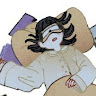Search
•Lena Du

# GAN Photo Editing

Project webpage

GitHub Repo (incoming)

#### B & W:

• Drew figures to explain the optimization process (in part1 and part2)

• Experiment with high-res models of Grumpy Cat

• Experiment with other datasets

### Part 1: Inverting the Generator

In proj3 and proj4, we have learned that:

• The Generator in GAN learns the features and can generate results that look like the training data from noise input.

• The process of style transfer could be done by extracting the (high-dimensional) features with a network using style loss and content loss. The Neural Style Transfer does not really need to train a network.

Therefore, the idea to invert the Generator is to get the feature that the Generator has learned. When we give the Generator an image, the Generator can find the (high-dimensional) features corresponding to this input image.

Then, the process could be explained by the picture below:Where the blue arrows indicate the part of Generator training in the GAN training process, the red arrows indicate how we use an inverted Generator to extract features. Note that generator G is already trained when we invert it.

Considering the process of training a generator G, we know that

y=G(x)

where x is the input image, y is the generated image. x could be another image or random noise. The way we estimate the performance of G is to compare y with its label, which is the real image.

We know that there are features z extracted from x by G, and it is the key for G to generate y. Then, what if we want to get z given a real image r such that we could generate any r-like result with any x? The answer is inverting G.

We could consider the process of getting z as an optimization problem:

z∗=arg⁡minzL(G(z),r)

where r is the real image.

#### Results:

The calculation of loss is

L=wL1∗L1+wperc∗Lperc

part 1.1 is comparing the result with different wperc

##### 1.1 Compare perceptual loss weights:
• latent space: z

• model: Style GAN

We can observe that the result varies when the perceptual loss changes. The result with weight0.0001 and 0.1 is better in this case

##### 1.2 Compare different models:
• latent space: z

• iteration number: 750

It is very obvious that the result using Style GAN has better quality, in terms of resolution, contrast, and color. Although the result using Vanilla GAN also maintains the basic structure of the original image.

##### 1.3 Compare latent spaces:
• model: Style GAN

• iteration number: 1000

With Style GAN, the results with 3 latent spaces are all good. But if we observe in detail, the results with latent space w and w+ have color better match the original image; the result with latent space w+ has the best accuracy of color and shape compared to the ones with z and w. However, the w+ has a lack of contrast, which could be considered caused by the mean operation.

### Part 2: Interpolate your Cats

The process of interpolation is very intuitive, though I still made some figures to demonstrate it:The first step is to get the features of the 2 images which are going to be interpolated:

z1=G−1(Image1)z2=G−1(Image2)

Note that the process to get the feature of the image with a generated is implemented by optimization as mentioned in part1.

We obtain the features of the 2 images with 2 optimizations:

z1=arg⁡minz1L(G(z1),Image1)z2=arg⁡minz2L(G(z2),Image2)

Then we will use the general interpolation method, which is

z′=θ1z1+θ2z2

For this case,

θ1=θθ2=1−θ

Therefore,

z′=θz1+(1−θ)z2

Then, we only need to generate the result with the combined feature z' by G:That is,

Combined Image=G(z′)

#### Results:

SourceDestinationTransition

This transition not only shows the face transiting, but it also shows how the color is interpolated in the transition. It is very smooth.

SourceDestinationTransition

The source and the destination images, in this case, are quite similar, thus the transition is harder to observe. However, if we compare the first frame with the last frame, we could find there is a clear difference and the transition is successful.

SourceDestinationTransition

This case shows how a cat's face is moving far away, there is also a color transition during the process.

### Part 3: Scribble to Image

Recall project 2 - Gradient Domain Fusion, the method we used to do image blending is optimization. To generate grumpy cats from scribble, we can also utilize this method, solving a soft-constrained optimization:

z∗=arg⁡minz∑i||fi(G(z))−vi||1

Here the v_i is the pixel in the given sketch.

However, we need to use masks because we are only concerned with the area that has a stroke in the sketch, and we can also reduce the equation under this situation to:

z∗=arg⁡minz||M∗fi(G(z))−M∗S||1

where M is the mask and S is the sketch.

#### Results:

We can see the facing direction, the angle, and the shape of the mouth are well generated according to the sketch.

### Bells & Whistles

#### - Experiment with high-res models

There are several sections that need to be adjusted for this experiment, here is an example of how to do the change:

```
name.startswith('stylegan') # originally: name == 'stylegan'

```

The command line to run this experiment should include

```
--model style256 --resolution 256

```

or

```
--model style128 --resolution 128

```

We can observe that the results with latent space w have nice details, for example, the reflection in the eyes and the cat whiskers. The results with latent space w+ have less high-contrast detail but the color and shape of the reconstruction are very accurate. Comparably, the results with latent space z are less good.

#### - Experiment with other datasets

Since the generator G is trained with the grumpy cat dataset, whatever input using this Generator to do the optimization will obtain a result that is close to a grumpy cat :)

It is fun that we could transfer any cat image to a grumpy cat image. Yay!